# PowerShell - Begin Process End

Written on June 24, 2018

If you look for or have used PowerShell scripts from the internet, you have likely encountered functions with the Begin, Process, and End blocks.

I did not really understand why these were useful until I ran into problems when trying to use the pipeline in my own functions. I will create 2 basic functions to illustrate the problem and solution. If you want to use the pipeline in PowerShell (and why wouldn't you); then you should be comfortable using these code blocks in your functions.

### In the Beginning, there was no Process or End

``````Function Test-NoBPE {
Param(
[Parameter(Position=0,ValueFromPipeline=\$true)]
)
}
``````

NOTE: In the Param block, I have set the add param to accept it’s value from the pipeline.
This useless function will add a number you choose to 1.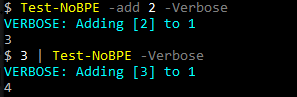### The Problem

It is all well and good when piping 1 value, but you run into a weird problem when using multiple values.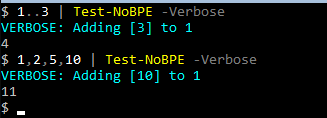Only the last piped value gets passed through properly. All prior values are lost.

### The Solution: Begin, Process, End

``````Function Test-BPE {
Param(
[Parameter(Position=0,ValueFromPipeline=\$true)]
)
Begin{
Write-Verbose "BEGIN"
}
Process{
}
End{
Write-Verbose "END"
}
}
``````

NOTE: Again, ValueFromPipeine is true, allowing the add param to get input from the pipeline.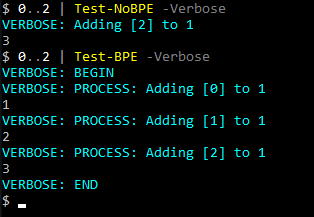Much better. As you can see, the Process block is acting more-or-less like a Foreach loop. Begin runs once, and End runs once. Process will iterate over all the input values. We can utilize the Begin and End blocks for setup and cleanup (in that order), or for other use cases.

#### Example

``````Function Test-BPE2 {
Param(
[Parameter(Position=0,ValueFromPipeline=\$true)]
)
Begin{
[int]\$total=0
Write-Verbose "BEGIN: Total=[\$total]"
}
Process{
}
End{
Write-Verbose "END"
Write-Output \$total
}
}
``````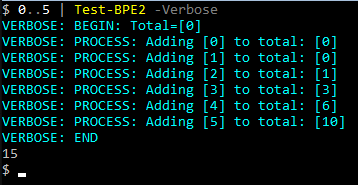You can use the Begin block to define a value or an empty list, then populate it in the Process block, and finally write the output in the End.

This implementation however may not be the best for sending output through the pipline. It certainly has its use cases; but if you want your functions to play nice with the pipline, it is better to write output as it is generated. This will allow the output to be fed through the pipeline as it goes, instead accumulating all the data and sending it over in one shot.

### A More Practical Example

``````Function Get-LDAPUser {
Param(
[Parameter(Position=0,Mandatory=\$true,ValueFromPipeline=\$true)]

[Parameter(Position=1)]
[String]\$orgUnit,

[Parameter(Position=2)]
[String[]]\$properties,

[Parameter(Position=3)]
[ValidateRange(0,2)]
[int16]\$scope=2
)
Begin{
\$searcher=New-Object -TypeName DirectoryServices.DirectorySearcher
if(!\$orgUnit){
\$rootDSE=New-Object -TypeName DirectoryServices.DirectoryEntry `
-ArgumentList 'LDAP://rootDSE'
\$orgUnit=\$rootDSE.Properties['defaultNamingContext'].Value
}
Write-Verbose "Searching under OU: [\$orgUnit]"
\$searchRoot=New-Object -TypeName DirectoryServices.DirectoryEntry `
-ArgumentList "LDAP://\$orgUnit"
\$searcher.SearchRoot=\$searchRoot
\$searcher.SearchScope=\$scope
if(\$PSBoundParameters.ContainsKey('properties')){
foreach(\$p in \$properties){
}
}
}
Process{
\$result=\$searcher.FindOne()
if(\$result){
\$result.GetDirectoryEntry()
}
}
End{
Write-Verbose 'Disposing of Directory Searcher'
\$searcher.Dispose()
}
}
``````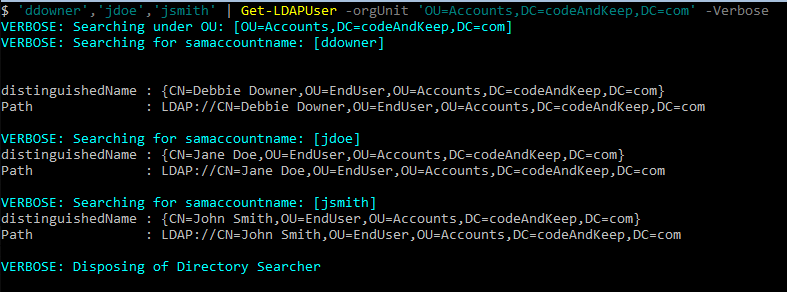We use the Begin block to setup our objects (directory searcher), then use the Process block to loop through our input using the object created above. We are writing the output as we go to ensure that this function will work well if we wanted to pipe this function to another function.

Finally we cleanup that object to avoid a memory leak (search for Directory Searcher memory leak for more info).